# CLASS 10 MATH NCERT SOLUTIONS FOR CHAPTER – 13 SURFACE AREAS AND VOLUMES EX – 13.2

## Surface Areas and Volumes

[Unless stated otherwise, take π = ]

Question 1.
A solid is in the shape of a cone standing on a hemisphere with both their radii being equal to 1 cm and the height of the cone is equal to its radius. Find the volume of the solid in terms of π.

Solution:
Radius of hemisphere and cone, r = 1 cm

Question 2.
Rachel, an engineering student, was asked to make a model shaped like a cylinder with two cones attached at its two ends by using a thin aluminium sheet. The diameter of the model is 3 cm and its length is 12 cm. If each cone has a height of 2 cm, find the volume of air contained in the model that Rachel made. (Assume the outer and inner dimensions of the model to be nearly the same.)

Solution:Question 3.
A gulab jamun, contains sugar syrup up to about 30% of its volume. Find approximately how much syrup would be found in 45 gulab jamuns, each shaped like a cylinder with two hemispherical ends with length 5 cm and diameter 2.8 cm (see figure).

Solution:
Radius of hemisphere part = 1.4 cm
Length of cylindrical part = 5 – 2.8 = 2.2 cm and its radius = 1.4 cm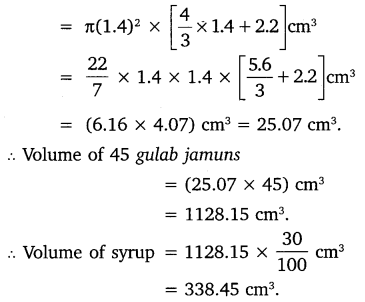Hence, there would be approximately 338 cm3 syrup in 45 gulab jamuns.

Question 4.
A pen stand made of wood is in the shape of a cuboid with four conical depressions to hold pens. The dimensions of the cuboid are 15 cm by 10 cm by 3.5 cm. The radius of each of the depressions is 0.5 cm and the depth is 1.4 cm. Find the volume of wood in the entire stand (see figure).

Solution:
Radius of conical hole, r = 0.5 cm
Its depth(height), h = 1.4 cm
Volume of wood = volume of conical part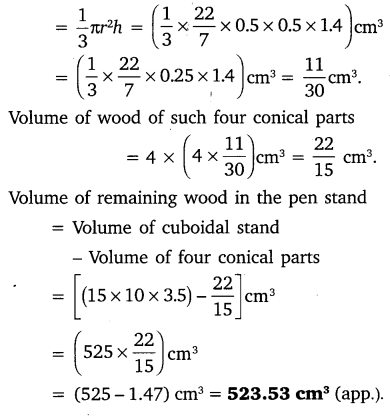Question 5.
A vessel is in the form of an inverted cone. Its height is 8 cm and the radius of its top, which is open, is 5 cm. It is filled with water up to the brim. When lead shots, each of which is a sphere of radius 0.5 cm are dropped into the vessel, one-fourth of the water flows out. Find the number of lead shots dropped in the vessel.

Solution:
Radius of cone, r = 5 cm
Height of cone, h = 8 cm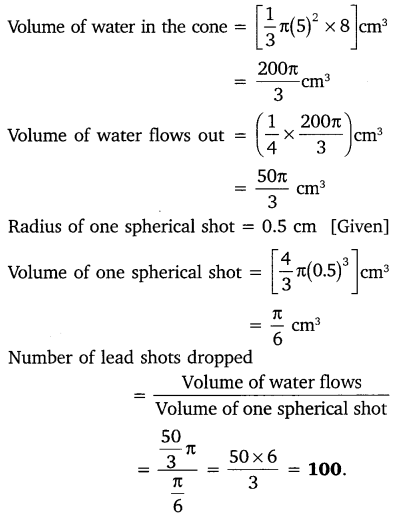Question 6.
A solid iron pole consists of a cylinder of height 220 cm and base diameter 24 cm, which is surmounted by another cylinder of height 60 cm and radius 8 cm. Find the mass of the pole, given that 1 cm3 of iron has approximately 8 g mass. (Use π = 3.14)

Solution:
Radius of first cylindrical part, r1 = 12 cm
Height of first cylindrical part, h1 = 220 cmRadius of second cylindrical part, r2 = 8 cm
Height of second cylindrical part, h2 = 60 cm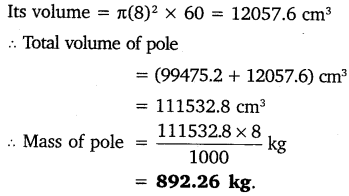Question 7.
A solid consisting of a right circular cone of height 120 cm and radius 60 cm standing on a hemisphere of radius 60 cm is placed upright in a right circular cylinder full of water such that it touches the bottom. Find the volume of water left in the cylinder, if the radius of the cylinder is 60 cm and its height is 180 cm.

Solution:
Radius of cylinder, R = 60 cm
Height of cylinder, H = 180 cm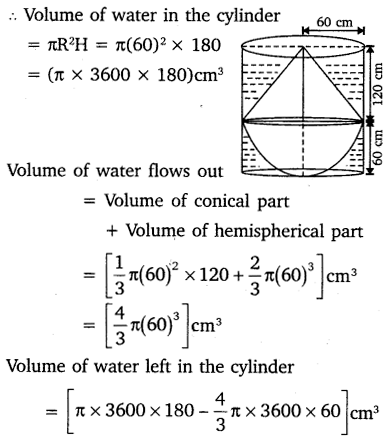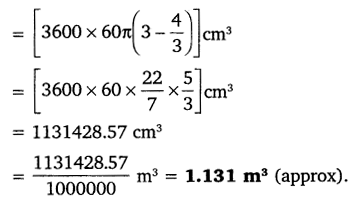Question 8.
A spherical glass vessel has a cylindrical neck 8 cm long, 2 cm in diameter, the diameter of the spherical part is 8.5 cm. By measuring the amount of water it holds, a child finds its volume to be 345 cm3. Check whether she is correct, taking the above as the inside measurements, and π = 3.14.

Solution:
Radius of cylinder part, r = 1 cm
Height of cylinder part, h = 8 cm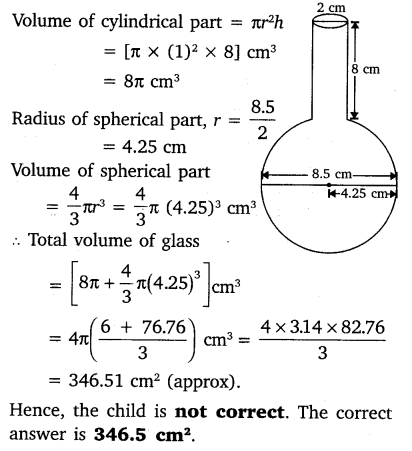### Take Your IIT JEE Coaching to Next Level with Examtube

• Mentoring & Teaching by IITians
• Regular Testing & Analysis
• Preparation for Various Engineering Entrance Exams
• Support for School/Board Exams
• 24/7 Doubts Clarification